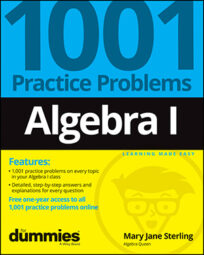##### Algebra I: 1001 Practice Problems For Dummies (+ Free Online Practice)Algebra problems are easier to solve when you know the rules and formulas. Unlike other subjects where you can just read or listen and absorb the information sufficiently, math takes practice.

The only way to figure out how the different algebraic rules work and interact with one another is to get into the problems and get your hands dirty, so to speak. Be prepared to practice on lots of different problems.

## Factoring in algebra

Factoring algebraic expressions is one of the most important techniques you need to practice. Not much else can be done in terms of solving equations, graphing functions and conics, and working on math applications if you can’t pull out a common factor and simplify an expression.

Here are some tips for factoring in algebra:

• Make sure you apply divisibility rules correctly.

• Write a prime factorization with the correct exponents on the prime factors.

• Check that the terms divided after dividing out a greatest common factor (GCF) don’t still have a common factor.

• Reduce only factors, not terms.

• Write fractional answers with correct grouping symbols to distinguish remaining factors.

### Factoring binomials

A binomial is an expression with two terms. The terms can be separated by addition or subtraction. You have four possibilities for factoring binomials:

• Factor out a greatest common factor.

• Factor as the difference of perfect squares.

• Factor as the difference of perfect cubes.

• Factor as the sum of perfect cubes.

If one of these methods doesn’t work, then the binomial doesn’t factor by using real numbers.

You can factor trinomials with the form ax2 + bx + c in one of two ways:

• Factor out a greatest common factor.

• Find two binomials whose product is that trinomial.

When finding the two binomials whose product is a particular trinomial, you work from the factors of the constant term and the factors of the coefficient of the lead term to create a sum or difference that matches the coefficient of the middle term. This technique can be expanded to trinomials that have the same general format but with exponents that are multiples of the basic trinomial.

## The basics of solving algebra equations

One of the most common goals in algebra I is solve an equation. Solving an equation means to identify the number or numbers you can replace the variable with to make a true statement. You’ll find factoring and the multiplication property of zero to be your first approach, and then you’ll also have the quadratic formula to use on some of the more challenging second degree equations. Polynomials can be solved using synthetic division to help with the factoring.

### Linear equations

Linear equations are of the form ax + b = c, where x is some variable, and a, b, and c are real numbers. To solve a linear equation, you perform a series of opposites:

• If a number is added to the term containing x, you subtract that number from both sides of the equation.

• If a number is subtracted from the term containing the variable, you add.

• If a number multiplies the variable, you divide.

• If a number divides the variable, you multiply.

Just be sure that whatever you do to one side of the equation, you also do to the other side. Think of the equation as two expressions pivoting on either side of a balance scale: You need to keep the sides at the same weight.

A quadratic equation has the form ax2 + bx + c = 0. The equation can have two solutions, only one solution (a double root), or no solutions among the real numbers. Where no real solution occurs, imaginary numbers are brought into the picture. Quadratic equations are solved most easily when the trinomial factors, but the quadratic formula is also a nice means to finding solutions.

### Polynomials

A polynomial is a smooth curve that goes on and on forever, from negative infinity to positive infinity. To solve a polynomial means to set the equation equal to 0 and determine which, if any, numbers create a true statement. Any numbers satisfying this equation give you important information: They tell you where the graph of the polynomial crosses or touches the xaxis.